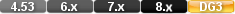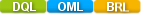Easy to Create, Easy to Change - Easy to use!## FutureValueFutureValue(PRESENT VALUE,INSTALLMENT,RATE,PERIODS) FutureValue(NUMERICAL VALUE,NUMERICAL VALUE,NUMERICAL VALUE,NUMERICAL VALUE)

The futurevalue function calculates the final value of a financial transaction given the presentvalue, installment payment, interest rate, and number of payment periods.

If the installment payment increases the value of the investment (e.g., an annuity), the installment must be expressed as a positive amount. If the payment decreases the value of the investment (e.g., loan amortization), the installment must be expressed as a negative amount.

The interest rate, installment payments, and time periods must be based on the same terms. For example, if payments are made monthly on a five-year loan, the number of periods is (12*5) or 60. The interest rate must be expressed in the same terms (the monthly interest rate is the annual rate divided by 12). The interest rate must also be expressed as a whole number (10% is 10, not 0.10).

# Parameters

PRESENT VALUE

The value at the start of the calculation. Ex. the amount borrowed.

INSTALLMENT

Postive value for Annuity (payment increase value) or Negative with Amortization (payment decrease the value)

RATE

The interest paid.

PERIODS

The number of periods

# Returns/Result

A numeric value (the final value after all payments are made).

# Examples

Example 1

futurevalue( 10000, 100, 10 / 12, 120)

Returns: 47,554.91

This example calculates the futurevalue (accumulated balance) of a monthly savings plan, if you start with \$10,000 and add \$100 every month for 12 years at an annual interest rate of 10%.

Example 2

futurevalue( 10000, 1200, 10, 12)

Returns: 57,045.42

This example calculates the futurevalue (accumulated balance) of an annual savings plan, starting with \$10,000 and adding \$1200 each year for 12 years at an annual interest rate of 10%. Both examples assume that installments are paid at the start of each period.

Note: When typing fractions like 10 / 12, DataEase requires that you type a space before and after the / division symbol.

DataEase financial functions are derived from the formula shown below. (The double asterisks (meaning "raise to the power") cannot be used in a script).

futurevalue = principal * ((1 + (rate/100)) ** periods) + (installment/(rate/100)) * (((1 + (rate/100)) ** periods) - 1)

# futurevalue

#### Type

Financial Function

#### Purpose

The futurevalue function calculates the final value of a financial transaction given the presentvalue, installment payment, interest rate, and number of payment periods.

Syntax

futurevalue( presentvalue, installment, rate, periods)

Returns

A numeric value (the final value after all payments are made).

Usage

If the installment payment increases the value of the investment (e.g., an annuity), the installment must be expressed as a positive amount. If the payment decreases the value of the investment (e.g., loan amortization), the installment must be expressed as a negative amount.

The interest rate, installment payments, and time periods must be based on the same terms. For example, if payments are made monthly on a five-year loan, the number of periods is (12*5) or 60. The interest rate must be expressed in the same terms (the monthly interest rate is the annual rate divided by 12). The interest rate must also be expressed as a whole number (10% is 10, not 0.10).

Example 1

futurevalue( 10000, 100, 10 / 12, 120)

Returns: 47,554.91

This example calculates the futurevalue (accumulated balance) of a monthly savings plan, if you start with \$10,000 and add \$100 every month for 12 years at an annual interest rate of 10%.

#### Example 2

futurevalue( 10000, 1200, 10, 12)

Returns: 57,045.42

This example calculates the futurevalue (accumulated balance) of an annual savings plan, starting with \$10,000 and adding \$1200 each year for 12 years at an annual interest rate of 10%. Both examples assume that installments are paid at the start of each period.

Note: When typing fractions like 10 / 12, DataEase requires that you type a space before and after the / division symbol.

DataEase financial functions are derived from the formula shown below. (The double asterisks (meaning "raise to the power") cannot be used in a script).

futurevalue = principal * ((1 + (rate/100)) ** periods) + (installment/(rate/100)) * (((1 + (rate/100)) ** periods) - 1)